﻿ 高斯表面在互连线参数提取中的应用 Application of Gaussian Surface to the Parameter Extraction of Interconnection Line

Applied Physics
Vol.07 No.11(2017), Article ID:22828,7 pages
10.12677/APP.2017.711042

Application of Gaussian Surface to the Parameter Extraction of Interconnection Line

Baojun Chen, Yanjie Ju

School of Electrical and Information Engineering, Dalian Jiaotong University, Dalian Liaoning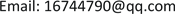Received: Nov. 4th, 2017; accepted: Nov. 17th, 2017; published: Nov. 28th, 2017ABSTRACT

With the further shrinkage of the feature size of integrated circuit, the reliability problem arising from RC delay of interconnection line becomes the main factor affecting the performance of chip. Affected by the manufacturing technology, the cross section of interconnection line is not regular rectangle, and RC delay is thus intensified. The analysis and computation of the parasitic parameter of such interconnection line using numerical method must firstly describe the rough surface. The surface height of interconnection line in the integrated circuit is not easy to measure. To this end, the paper proposes using Gaussian function to describe the rough surface. The experimental data indicate that the application of such surface to the parameters of interconnection line realizes accurate computation result.

Keywords:Gaussian Function, Interconnection Line, Parameter ExtractionCopyright © 2017 by authors and Hans Publishers Inc.

This work is licensed under the Creative Commons Attribution International License (CC BY).

http://creativecommons.org/licenses/by/4.0/1. 引言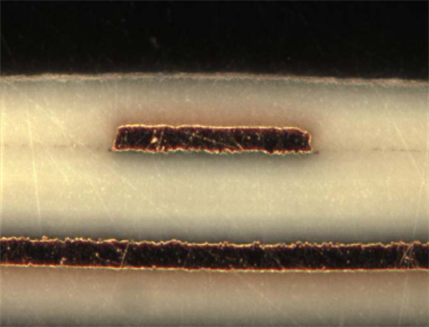Figure1. The surface of interconnect is rough

2. 生成高斯表面

$\overline{h}={〈h\left(x\right)〉}_{s}={\int }_{-\infty }^{\infty }hp\left(h\right)\text{d}h$ (1)

$〈\text{ }〉$ 表示沿整个粗糙面求高度的平均值，通常我们都选取适当的参考面，使得相对于此参考面的高度 $h\left(x\right)$ 的均值为零，这会给计算带来很大的方便。

$\text{RMS}=\sqrt{\frac{1}{\text{length}}{\int }_{0}^{\text{length}}{h}^{2}\left(x\right)\text{d}x}$ (2)

$C\left(l\right)=〈h\left(x\right)h\left(x+l\right)〉$ (3)

$\rho \left(l\right)=\frac{C\left(l\right)}{{\text{RMS}}^{\text{2}}}=\frac{〈h\left(x\right)h\left(x+l\right)〉}{{\text{RMS}}^{\text{2}}}$ (4)

$U=F\left(x\right)$ ，即可得：

$X={F}^{-1}\left(U\right)$ (5)

$U=X\mathrm{cos}\theta$ , $V=X\mathrm{sin}\theta$ (6)

$F\left(x\right)=\left\{\begin{array}{l}0\text{ }\text{ }\text{ }\text{ }\text{ }\text{ }\text{ }\text{ }\text{ }x<0\\ 1-\mathrm{exp}\left(-{x}^{2}/2{\sigma }^{2}\right)\text{ }\text{ }\text{\hspace{0.17em}}\text{\hspace{0.17em}}x\ge 0\end{array}$ (7)

$W=F\left(x\right)$ ，即可得

$X={F}^{-1}\left(W\right)=\sqrt{2{\sigma }^{2}\mathrm{ln}\left(\frac{1}{1-W}\right)}$ (8)

3. 粗糙表面参数计算结果

${\sigma }_{\text{discrepancy}}=\frac{\left({\sigma }_{\text{rough}}-{\sigma }_{\text{smooth}}\right)}{{\sigma }_{\text{smooth}}}$ (9)(a)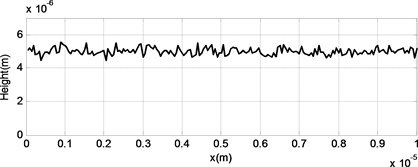(b)(c)

Figure 2. Gaussian rough surface (a) RMS = 0.5 um, (b) RMS = 0.2 um, (c) RMS = 0.1 um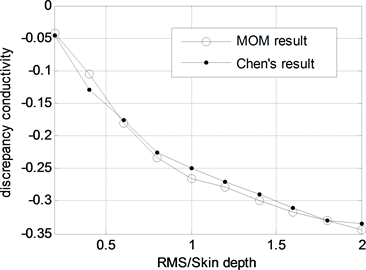Figure 3. Corresponding discrepancy between mean effective conductivity and normal conductivity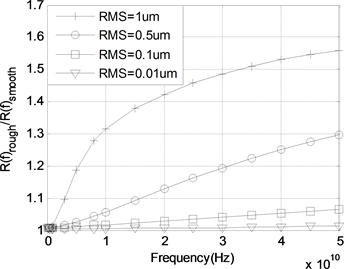Figure 4. Resistance ratio as a function of frequency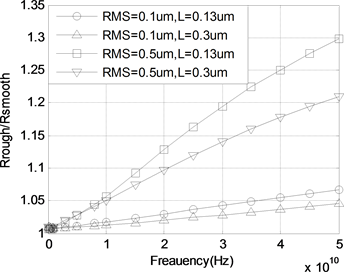Figure 5. Resistance ratio as a function of frequency

4. 结论

Application of Gaussian Surface to the Parameter Extraction of Interconnection Line[J]. 应用物理, 2017, 07(11): 344-350. http://dx.doi.org/10.12677/APP.2017.711042

1. 1. Wu, Z. and Davis, L.E. (1994) Surface Roughness Effect on Surface of Impedance of Superconductors. Journal of Applied Physics, 76, 3669-3672. https://doi.org/10.1063/1.357430

2. 2. Biot, M.A. (1957) Some New Aspects of the Reflection of Electromagnetic Waves on a Rough Surface. Journal of Applied Physics, 28, 1455-1463. https://doi.org/10.1063/1.1722676

3. 3. Wait, J.R. (1959) Guiding of Electromagnetic Waves by Uniformly Rough Surface-Part 1. Antennas and Propagation, 7, 154-162. https://doi.org/10.1109/TAP.1959.1144764

4. 4. Zhu, Z.H., Demir, A. and White, J.B. (2004) A Stochastic Integral Equation Method for Modeling the Rough Surface Effect On Interconnect Capacitance. Computer Aided Design, 887-891.

5. 5. Wilton, D., Rao, S. and Glisson, A. (1984) Potential Integrals for Uniform and Linear Source Distributions on Polygonal and Polyhedral Domains. IEEE Transactions on Antennas and Propagation, 32, 276-281. https://doi.org/10.1109/TAP.1984.1143304

6. 6. Gralia, R.D. (1993) On the Numerical Integration of the Linear Shape Functions Times the 3-D Greens Function or Its Gradient on a Place Triangle. IEEE Transactions on Antennas and Propagation, 41, 1448-1455. https://doi.org/10.1109/8.247786

7. 7. Morgan, S.P. and Samuel, P. (1949) Effect of Surface Roughness on Eddy Current Losses at Microwave Frequencies. Applied Physics, 20, 352-362. https://doi.org/10.1063/1.1698368

8. 8. 盛新庆. 计算电磁学要论[M]. 北京: 科学出版社, 2004.

9. 9. Duffy, M.G. (1982) Quadrature over a Pyramid or Cube of Integrands with a Singularity at a Vertex. SIAM Journal on Numerical Analysis, 19, 1260-1262. https://doi.org/10.1137/0719090

10. 10. 王浩刚, 聂在平. 三维矢量散射积分方程中奇异性分析[J]. 电子学报, 1999, 27(12): 68-71.

11. 11. Geoffrey, R.G. and David, R.S. (2001) Probability and Random Processes. Oxford University Press, Oxford.

12. 12. Tsang, L., Kong, J.A. (2001) Electromagnetic Waves: Numerical Simulations. John Wiley and Sins, New York. https://doi.org/10.1002/0471224308

13. 13. Chen, Q. and Wong, N. (2008) Efficient Numerical Modeling of Random Rough Surface Effects for Interconnect Internal Impedance Extraction. Design Automation Conference.